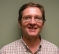## Filter complex signal and get spectraStarted by 5 years ago2 replieslatest reply 5 years ago132 views

How to correctly get spectrum of filtered complex signal in time domain

Filter with real coefficients (LPF). [1 2 1]The frequency response is in general a complex quantity, in most cases obeys certain symmetry properties. Сomplex signal not symmetry.

As can be seen from Fig., The spectra obtained shows when I filter the signal, the filter becomes not a LPF, but a band stop filter!!!Here the question, how correctly filter complex signal. And if I filter correctly, then how get correct spectrum of output signal.

To experiment try code below.

clear all
clc
Fs = 64;            % Sampling frequency
T = 1/Fs;             % Sampling period
L = 64;             % Length of signal
t = (0:L-1)*T;
%% Init filter
b = [1 2 1];    % FIR coef
h = freqz(b,1, L, 'twosided');
figure(1)
plot(abs(h))
title('Frequency responce of LOWPASS filter')
%% Init signal
f = 32; %freq
x = exp(j*2*pi * f.*t);
re = real(x);
im = imag(x);
%% Process filtering in time domain
s = filter(b,1,x);
s_re = real(s);
s_im = imag(s);
figure(2)
subplot(4,1,1)
plot(re)
title('In-Phase input signal')
subplot(4,1,2)
plot(im)
figure(2)
subplot(4,1,3)
title('In-Phase output signal')
plot(s_re)
subplot(4,1,4)
plot(s_im)
%% Get spectra one way
figure(3)
subplot(2,1,1)
plot(abs(fft(x))/L)
title('Input signal spectrum')
subplot(2,1,2)
plot(abs(fft(s))/L)
title('Output signal spectrum')
%% Or another way
% Get spectrum in each component
spec_x_re = abs(fft(re))/L;
spec_x_im = abs(fft(im))/L;
spec_s_re = abs(fft(s_re))/L;
spec_s_im = abs(fft(s_im))/L;
figure(4)
subplot(4,1,1)
plot(spec_x_re)
subplot(4,1,2)
plot(spec_x_im)
subplot(4,1,3)
plot(spec_s_re)
subplot(4,1,4)
plot(spec_s_im)

[ - ]Hello,

Ran you code and looked at the output.

Problem is that you are looking at the spectrum of the startup transient of an extremely short filter with a high frequency signal, Fs/4.

Cut off the points in the beginning.

Cheers,

Mark Napier

[ - ]